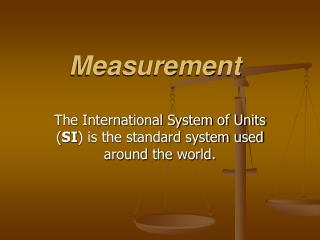DownloadDownload PresentationMeasurement

# Measurement

Download Presentation## Measurement

- - - - - - - - - - - - - - - - - - - - - - - - - - - E N D - - - - - - - - - - - - - - - - - - - - - - - - - - -
##### Presentation Transcript

1. Measurement The International System of Units (SI) is the standard system used around the world.

2. Length • The standard SI unit to measure length is the meter (m). • The meter is divided into 100 equal parts called centimeters (cm). • The centimeter is divided into 10 equal parts called millimeters (mm). • Long distances are measured in kilometers (km) which is 1,000 m.

3. Mass • Mass is the amount of matter that something is made of. • The kilogram is the SI unit to measure mass. • Mass is measured with a triple balance beam. • Unlike weight, mass does not change with an object’s position.

4. Weight • Weight is a measurement of the gravitational force on an object. • The SI unit for force is the Newton (N). • Since weight is a measure of gravitational force, it does matter where you are. • There is 6 times less gravitational force on the moon, so you would weight 6 times less there. • Use spring scales to measure weight.

5. Density • Density is mass per unit volume. • Density is the amount of matter it has in a given space. • To find density (D), first measure the mass (m) and volume (V), then divide mass by volume. • Density is an SI derived unit (mass and volume)

6. Volume • Volume is the amount of space that something occupies. • Volumes of liquids are expressed as liters (L). • Graduated cylinders are used to measure liquid volume, and also to find the volume of a small odd shaped object. (see meniscus) • Volumes of solid objects are expressed in cubic meters (cm3)

7. Measurement • Area is the amount of surface included within a set of boundaries and is expressed in square such as square meters (m2). • The amount of space occupied by an object is the object’s volume. Area and Volume • The basic SI unit of volume for a solid object is the cubic meter (m3). • SI measurements for liquid volumes are in milliliters (mL) or liters (L).

8. Temperature • Temperature is a measure of the average kinetic energy of the particles in an object. • Temperature is not determined by how much of the substance you have. • The SI unit for temperature is Kelvin (K), however the Celsius (C) scale is most commonly used.